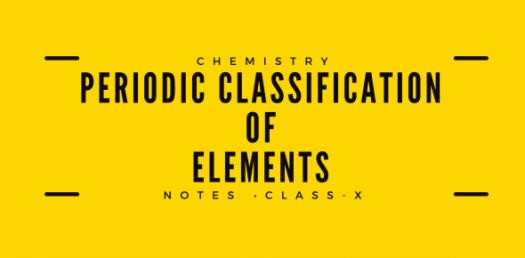# Chemistry Quiz: Periodic Classification Of Elements

15 Questions | Attempts: 6120Settings.

• 1.
Which of the following is not true for metalloids?
• A.

They are borderline elements that exhibit both metallic and non metallic properties to some extent

• B.

They usually act as electron donors with non-metals and as electron acceptors with metals

• C.

Some of these elements are boron, silicon and germanium

• D.

They are good conductors of heat and electricity

• 2.
Six elements A, B, C, D, E and F have the following atomic numbers (A = 12, B = 17, C = 18, D = 7, E = 9 and F = 11). Among these elements, the element, which belongs to the 3rd period and has the highest ionization potential, is
• A.

A

• B.

B

• C.

C

• D.

F

• 3.
Which of the following are the characteristics of isotopes of an element?       i. Isotopes of an element have same atomic masses     ii. Isotopes of an element have same atomic number     iii. Isotopes of an element show same physical properties     iv. Isotopes of an element show same chemical properties
• A.

(i), (iii) and (iv)

• B.

(ii), (iii) and (iv)

• C.

(ii) and (iii)

• D.

(ii) and (iv)

• 4.
Arrange the following elements in the order of their increasing nonmetallic character: Li, O, C, Be, F
• A.

F < O < C < Be < Li

• B.

Li < Be < C < O< F

• C.

F < O < C < Be < Li

• D.

F < O < Be < C < Li

• 5.
Which one of the following depicts the correct representation of atomic radius(r) of an atom?
• A.

(i) and (ii)

• B.

(ii) and (iii)

• C.

(iii) and (iv)

• D.

(i) and (iv)

• 6.
Which of the following hydroxides is most basic?
• A.

Be(OH)2

• B.

Ba(OH)2

• C.

Ca(OH)2

• D.

Mg(OH)2

• 7.
Which of the following is Dobereiner’s triad?
• A.

Ne,Ca,Na

• B.

H2,N2, O2

• C.

Li, Na, K

• D.

Na, Br, Ar

• 8.
The statement that is not true about electron affinity is
• A.

It causes energy to be released

• B.

It causes energy to be absorbed

• C.

It is expressed in electron volts

• D.

It involves formation of an anion

• 9.
Elements in the same vertical group of the periodic table have same
• A.

Atomic number

• B.

Atomic volume

• C.

Number of valence electrons

• D.

Atomic mass

• 10.
Identify the p-block elements which are not representative elements?
• A.

I-A

• B.

IV-A

• C.

VII-A

• D.

VIII-A

• 11.
Identify the incorrect statement
• A.

All the metals are good conductor of electricity

• B.

All the metals are good conductor of heat

• C.

All the metals form positive ions

• D.

All the metals form acidic oxides

• 12.
The first ionization energy of an element is 736 kJ per mole of atoms. An atom of this element in the ground state has a total of how many valence electrons?
• A.

1

• B.

2

• C.

3

• D.

4

• 13.
Which of the following is the correct order of decreasing first ionization energy:
• A.

C > B > Be > Li

• B.

C > Be > B > Li

• C.

B > C > Be > Li

• D.

Be > Li > B > C

• 14.
Which of the following statement (s) about the Modern Periodic Table are incorrect       i. The elements in the Modern Periodic Table are arranged on the basis of their decreasing atomic number       ii. The elements in the Modern Periodic Table are arranged on the basis of their increasing atomic masses       iii. Isotopes are placed in adjoining group (s) in the Periodic Table       iv. The elements in the Modern Periodic Table are arranged on the basis of their increasing atomic number
• A.

(i) only

• B.

(i), (ii) and (iii)

• C.

(i), (ii) and (iv)

• D.

(iv) only

• 15.
For a given value of ‘n’, the ionization energy is highest for:
• A.

D-electrons

• B.

F-electrons

• C.

P-electrons

• D.

S-electrons

## Related TopicsBack to top
×

Wait!
Here's an interesting quiz for you.